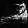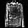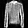» Code examples / Generative Deep Learning / WGAN-GP overriding `Model.train_step`

# WGAN-GP overriding `Model.train_step`

Author: A_K_Nain
Date created: 2020/05/9
Description: Implementation of Wasserstein GAN with Gradient Penalty.View in ColabGitHub source

## Wasserstein GAN (WGAN) with Gradient Penalty (GP)

The original Wasserstein GAN leverages the Wasserstein distance to produce a value function that has better theoretical properties than the value function used in the original GAN paper. WGAN requires that the discriminator (aka the critic) lie within the space of 1-Lipschitz functions. The authors proposed the idea of weight clipping to achieve this constraint. Though weight clipping works, it can be a problematic way to enforce 1-Lipschitz constraint and can cause undesirable behavior, e.g. a very deep WGAN discriminator (critic) often fails to converge.

WGAN-GP proposed an alternative to weight clipping to ensure smooth training. Instead of clipping the weights, the authors proposed a "gradient penalty": adding a loss term that keeps the L2 norm of the discriminator gradients close to 1.

## Setup

``````import numpy as np
import tensorflow as tf
from tensorflow import keras
from tensorflow.keras import layers
``````

## Prepare Fashion-MNIST data

We will be using the Fashion-MNIST dataset in this example to demonstrate the training of WGAN-GP. Each sample in this dataset is a 28x28 grayscale image associated with a label from 10 classes (e.g. Trouser, Pullover, Sneaker, etc.)

``````IMG_SHAPE = (28, 28, 1)
BATCH_SIZE = 512

# Size of noise vector
noise_dim = 128

fashion_mnist = keras.datasets.fashion_mnist
(train_images, train_labels), (test_images, test_labels) = fashion_mnist.load_data()
print(f"Number of examples: {len(train_images)}")
print(f"Shape of the images in the dataset: {train_images.shape[1:]}")

# we will reshape each sample to (28, 28, 1) and normalize the pixel values in [-1, 1].
train_images = train_images.reshape(train_images.shape, *IMG_SHAPE).astype("float32")
train_images = (train_images - 127.5) / 127.5
``````
``````Number of examples: 60000
Shape of the images in the dataset: (28, 28)
``````

## Create the discriminator (aka critic in the original WGAN)

The samples in the dataset have shape `(28, 28, 1)`. As we will be using strided convolutions, this can result in a shape with odd dimensions. For example, `(28, 28) -> Conv_s2 -> (14, 14) -> Conv_s2 -> (7, 7) -> Conv_s2 ->(3, 3)`.

While doing upsampling in the generator, we won't get the same input shape as the original images if we aren't careful. To avoid this, we will do something much simpler. In the discriminator, we will "zero pad" the input to make the shape `(32, 32, 1)` for each sample, while in the generator we will crop the final output to match the shape with input shape.

``````def conv_block(
x,
filters,
activation,
kernel_size=(3, 3),
strides=(1, 1),
use_bias=True,
use_bn=False,
use_dropout=False,
drop_value=0.5,
):
x = layers.Conv2D(
)(x)
if use_bn:
x = layers.BatchNormalization()(x)
x = activation(x)
if use_dropout:
x = layers.Dropout(drop_value)(x)
return x

def get_discriminator_model():
img_input = layers.Input(shape=IMG_SHAPE)
# Zero pad the input to make the input images size to (32, 32, 1).
x = conv_block(
x,
64,
kernel_size=(5, 5),
strides=(2, 2),
use_bn=False,
use_bias=True,
activation=layers.LeakyReLU(0.2),
use_dropout=False,
drop_value=0.3,
)
x = conv_block(
x,
128,
kernel_size=(5, 5),
strides=(2, 2),
use_bn=False,
activation=layers.LeakyReLU(0.2),
use_bias=True,
use_dropout=True,
drop_value=0.3,
)
x = conv_block(
x,
256,
kernel_size=(5, 5),
strides=(2, 2),
use_bn=False,
activation=layers.LeakyReLU(0.2),
use_bias=True,
use_dropout=True,
drop_value=0.3,
)
x = conv_block(
x,
512,
kernel_size=(5, 5),
strides=(2, 2),
use_bn=False,
activation=layers.LeakyReLU(0.2),
use_bias=True,
use_dropout=False,
drop_value=0.3,
)

x = layers.Flatten()(x)
x = layers.Dropout(0.2)(x)
x = layers.Dense(1)(x)

d_model = keras.models.Model(img_input, x, name="discriminator")
return d_model

d_model = get_discriminator_model()
d_model.summary()
``````
``````Model: "discriminator"
_________________________________________________________________
Layer (type)                 Output Shape              Param #
=================================================================
input_1 (InputLayer)         [(None, 28, 28, 1)]       0
_________________________________________________________________
_________________________________________________________________
conv2d (Conv2D)              (None, 16, 16, 64)        1664
_________________________________________________________________
leaky_re_lu (LeakyReLU)      (None, 16, 16, 64)        0
_________________________________________________________________
conv2d_1 (Conv2D)            (None, 8, 8, 128)         204928
_________________________________________________________________
leaky_re_lu_1 (LeakyReLU)    (None, 8, 8, 128)         0
_________________________________________________________________
dropout (Dropout)            (None, 8, 8, 128)         0
_________________________________________________________________
conv2d_2 (Conv2D)            (None, 4, 4, 256)         819456
_________________________________________________________________
leaky_re_lu_2 (LeakyReLU)    (None, 4, 4, 256)         0
_________________________________________________________________
dropout_1 (Dropout)          (None, 4, 4, 256)         0
_________________________________________________________________
conv2d_3 (Conv2D)            (None, 2, 2, 512)         3277312
_________________________________________________________________
leaky_re_lu_3 (LeakyReLU)    (None, 2, 2, 512)         0
_________________________________________________________________
flatten (Flatten)            (None, 2048)              0
_________________________________________________________________
dropout_2 (Dropout)          (None, 2048)              0
_________________________________________________________________
dense (Dense)                (None, 1)                 2049
=================================================================
Total params: 4,305,409
Trainable params: 4,305,409
Non-trainable params: 0
_________________________________________________________________
``````

## Create the generator

``````def upsample_block(
x,
filters,
activation,
kernel_size=(3, 3),
strides=(1, 1),
up_size=(2, 2),
use_bn=False,
use_bias=True,
use_dropout=False,
drop_value=0.3,
):
x = layers.UpSampling2D(up_size)(x)
x = layers.Conv2D(
)(x)

if use_bn:
x = layers.BatchNormalization()(x)

if activation:
x = activation(x)
if use_dropout:
x = layers.Dropout(drop_value)(x)
return x

def get_generator_model():
noise = layers.Input(shape=(noise_dim,))
x = layers.Dense(4 * 4 * 256, use_bias=False)(noise)
x = layers.BatchNormalization()(x)
x = layers.LeakyReLU(0.2)(x)

x = layers.Reshape((4, 4, 256))(x)
x = upsample_block(
x,
128,
layers.LeakyReLU(0.2),
strides=(1, 1),
use_bias=False,
use_bn=True,
use_dropout=False,
)
x = upsample_block(
x,
64,
layers.LeakyReLU(0.2),
strides=(1, 1),
use_bias=False,
use_bn=True,
use_dropout=False,
)
x = upsample_block(
x, 1, layers.Activation("tanh"), strides=(1, 1), use_bias=False, use_bn=True
)
# At this point, we have an output which has the same shape as the input, (32, 32, 1).
# We will use a Cropping2D layer to make it (28, 28, 1).
x = layers.Cropping2D((2, 2))(x)

g_model = keras.models.Model(noise, x, name="generator")
return g_model

g_model = get_generator_model()
g_model.summary()
``````
``````Model: "generator"
_________________________________________________________________
Layer (type)                 Output Shape              Param #
=================================================================
input_2 (InputLayer)         [(None, 128)]             0
_________________________________________________________________
dense_1 (Dense)              (None, 4096)              524288
_________________________________________________________________
batch_normalization (BatchNo (None, 4096)              16384
_________________________________________________________________
leaky_re_lu_4 (LeakyReLU)    (None, 4096)              0
_________________________________________________________________
reshape (Reshape)            (None, 4, 4, 256)         0
_________________________________________________________________
up_sampling2d (UpSampling2D) (None, 8, 8, 256)         0
_________________________________________________________________
conv2d_4 (Conv2D)            (None, 8, 8, 128)         294912
_________________________________________________________________
batch_normalization_1 (Batch (None, 8, 8, 128)         512
_________________________________________________________________
leaky_re_lu_5 (LeakyReLU)    (None, 8, 8, 128)         0
_________________________________________________________________
up_sampling2d_1 (UpSampling2 (None, 16, 16, 128)       0
_________________________________________________________________
conv2d_5 (Conv2D)            (None, 16, 16, 64)        73728
_________________________________________________________________
batch_normalization_2 (Batch (None, 16, 16, 64)        256
_________________________________________________________________
leaky_re_lu_6 (LeakyReLU)    (None, 16, 16, 64)        0
_________________________________________________________________
up_sampling2d_2 (UpSampling2 (None, 32, 32, 64)        0
_________________________________________________________________
conv2d_6 (Conv2D)            (None, 32, 32, 1)         576
_________________________________________________________________
batch_normalization_3 (Batch (None, 32, 32, 1)         4
_________________________________________________________________
activation (Activation)      (None, 32, 32, 1)         0
_________________________________________________________________
cropping2d (Cropping2D)      (None, 28, 28, 1)         0
=================================================================
Total params: 910,660
Trainable params: 902,082
Non-trainable params: 8,578
_________________________________________________________________
``````

## Create a WGAN-GP model

Now that we have defined our generator and discriminator models, we will implement the WGAN-GP model. We will override the `train_step` for training.

``````class WGAN(keras.Model):
def __init__(
self,
discriminator,
generator,
latent_dim,
discriminator_extra_steps=3,
gp_weight=10.0,
):
super(WGAN, self).__init__()
self.discriminator = discriminator
self.generator = generator
self.latent_dim = latent_dim
self.d_steps = discriminator_extra_steps
self.gp_weight = gp_weight

def compile(self, d_optimizer, g_optimizer, d_loss_fn, g_loss_fn):
super(WGAN, self).compile()
self.d_optimizer = d_optimizer
self.g_optimizer = g_optimizer
self.d_loss_fn = d_loss_fn
self.g_loss_fn = g_loss_fn

This loss is calculated on an interpolated image
and added to the discriminator loss.
"""
# get the interplated image
alpha = tf.random.normal([batch_size, 1, 1, 1], 0.0, 1.0)
diff = fake_images - real_images
interpolated = real_images + alpha * diff

gp_tape.watch(interpolated)
# 1. Get the discriminator output for this interpolated image.
pred = self.discriminator(interpolated, training=True)

# 2. Calculate the gradients w.r.t to this interpolated image.
# 3. Calcuate the norm of the gradients
norm = tf.sqrt(tf.reduce_sum(tf.square(grads), axis=[1, 2, 3]))
gp = tf.reduce_mean((norm - 1.0) ** 2)
return gp

def train_step(self, real_images):
if isinstance(real_images, tuple):
real_images = real_images

# Get the batch size
batch_size = tf.shape(real_images)

# For each batch, we are going to perform the
# following steps as laid out in the original paper.
# 1. Train the generator and get the generator loss
# 2. Train the discriminator and get the discriminator loss
# 3. Calculate the gradient penalty
# 4. Multiply this gradient penalty with a constant weight factor
# 6. Return generator and discriminator losses as a loss dictionary.

# Train discriminator first. The original paper recommends training
# the discriminator for `x` more steps (typically 5) as compared to
# one step of the generator. Here we will train it for 3 extra steps
# as compared to 5 to reduce the training time.
for i in range(self.d_steps):
# Get the latent vector
random_latent_vectors = tf.random.normal(
shape=(batch_size, self.latent_dim)
)
# Generate fake images from the latent vector
fake_images = self.generator(random_latent_vectors, training=True)
# Get the logits for the fake images
fake_logits = self.discriminator(fake_images, training=True)
# Get the logits for real images
real_logits = self.discriminator(real_images, training=True)

# Calculate discriminator loss using fake and real logits
d_cost = self.d_loss_fn(real_img=real_logits, fake_img=fake_logits)
d_loss = d_cost + gp * self.gp_weight

# Get the gradients w.r.t the discriminator loss
# Update the weights of the discriminator using the discriminator optimizer
)

# Train the generator now.
# Get the latent vector
random_latent_vectors = tf.random.normal(shape=(batch_size, self.latent_dim))
# Generate fake images using the generator
generated_images = self.generator(random_latent_vectors, training=True)
# Get the discriminator logits for fake images
gen_img_logits = self.discriminator(generated_images, training=True)
# Calculate the generator loss
g_loss = self.g_loss_fn(gen_img_logits)

# Get the gradients w.r.t the generator loss
# Update the weights of the generator using the generator optimizer
)
return {"d_loss": d_loss, "g_loss": g_loss}
``````

## Create a callback that periodically saves generated images

``````class GANMonitor(keras.callbacks.Callback):
def __init__(self, num_img=6, latent_dim=128):
self.num_img = num_img
self.latent_dim = latent_dim

def on_epoch_end(self, epoch, logs=None):
random_latent_vectors = tf.random.normal(shape=(self.num_img, self.latent_dim))
generated_images = self.model.generator(random_latent_vectors)
generated_images = (generated_images * 127.5) + 127.5

for i in range(self.num_img):
img = generated_images[i].numpy()
img = keras.preprocessing.image.array_to_img(img)
img.save("generated_img_{i}_{epoch}.png".format(i=i, epoch=epoch))
``````

## Train the end-to-end model

``````# Optimizer for both the networks
# learning_rate=0.0002, beta_1=0.5 are recommened
learning_rate=0.0002, beta_1=0.5, beta_2=0.9
)
learning_rate=0.0002, beta_1=0.5, beta_2=0.9
)

# Define the loss functions to be used for discrimiator
# This should be (fake_loss - real_loss)
# We will add the gradient penalty later to this loss function
def discriminator_loss(real_img, fake_img):
real_loss = tf.reduce_mean(real_img)
fake_loss = tf.reduce_mean(fake_img)
return fake_loss - real_loss

# Define the loss functions to be used for generator
def generator_loss(fake_img):
return -tf.reduce_mean(fake_img)

# Epochs to train
epochs = 20

# Callbacks
cbk = GANMonitor(num_img=3, latent_dim=noise_dim)

# Get the wgan model
wgan = WGAN(
discriminator=d_model,
generator=g_model,
latent_dim=noise_dim,
discriminator_extra_steps=3,
)

# Compile the wgan model
wgan.compile(
d_optimizer=discriminator_optimizer,
g_optimizer=generator_optimizer,
g_loss_fn=generator_loss,
d_loss_fn=discriminator_loss,
)

# Start training
wgan.fit(train_images, batch_size=BATCH_SIZE, epochs=epochs, callbacks=[cbk])
``````
``````Epoch 1/20
118/118 [==============================] - 39s 334ms/step - d_loss: -7.6571 - g_loss: -16.9272
Epoch 2/20
118/118 [==============================] - 39s 334ms/step - d_loss: -7.2396 - g_loss: -8.5466
Epoch 3/20
118/118 [==============================] - 40s 335ms/step - d_loss: -6.3892 - g_loss: 1.3971
Epoch 4/20
118/118 [==============================] - 40s 335ms/step - d_loss: -5.7705 - g_loss: 6.5997
Epoch 5/20
118/118 [==============================] - 40s 336ms/step - d_loss: -5.2659 - g_loss: 7.4743
Epoch 6/20
118/118 [==============================] - 40s 335ms/step - d_loss: -4.9563 - g_loss: 6.2071
Epoch 7/20
118/118 [==============================] - 40s 335ms/step - d_loss: -4.5759 - g_loss: 6.4767
Epoch 8/20
118/118 [==============================] - 40s 335ms/step - d_loss: -4.3748 - g_loss: 5.4304
Epoch 9/20
118/118 [==============================] - 40s 335ms/step - d_loss: -4.1142 - g_loss: 6.4326
Epoch 10/20
118/118 [==============================] - 40s 335ms/step - d_loss: -3.7956 - g_loss: 7.1200
Epoch 11/20
118/118 [==============================] - 40s 335ms/step - d_loss: -3.5723 - g_loss: 7.1837
Epoch 12/20
118/118 [==============================] - 40s 335ms/step - d_loss: -3.4374 - g_loss: 9.0537
Epoch 13/20
118/118 [==============================] - 40s 335ms/step - d_loss: -3.3402 - g_loss: 8.4949
Epoch 14/20
118/118 [==============================] - 40s 335ms/step - d_loss: -3.1252 - g_loss: 8.6130
Epoch 15/20
118/118 [==============================] - 40s 336ms/step - d_loss: -3.0130 - g_loss: 9.4563
Epoch 16/20
118/118 [==============================] - 40s 335ms/step - d_loss: -2.9330 - g_loss: 8.8075
Epoch 17/20
118/118 [==============================] - 40s 336ms/step - d_loss: -2.7980 - g_loss: 8.0775
Epoch 18/20
118/118 [==============================] - 40s 335ms/step - d_loss: -2.7835 - g_loss: 8.7983
Epoch 19/20
118/118 [==============================] - 40s 335ms/step - d_loss: -2.6409 - g_loss: 7.8309
Epoch 20/20
118/118 [==============================] - 40s 336ms/step - d_loss: -2.5134 - g_loss: 8.6653

<tensorflow.python.keras.callbacks.History at 0x7fc1a410a278>
``````

Display the last generated images:

``````from IPython.display import Image, display

display(Image("generated_img_0_19.png"))
display(Image("generated_img_1_19.png"))
display(Image("generated_img_2_19.png"))
``````